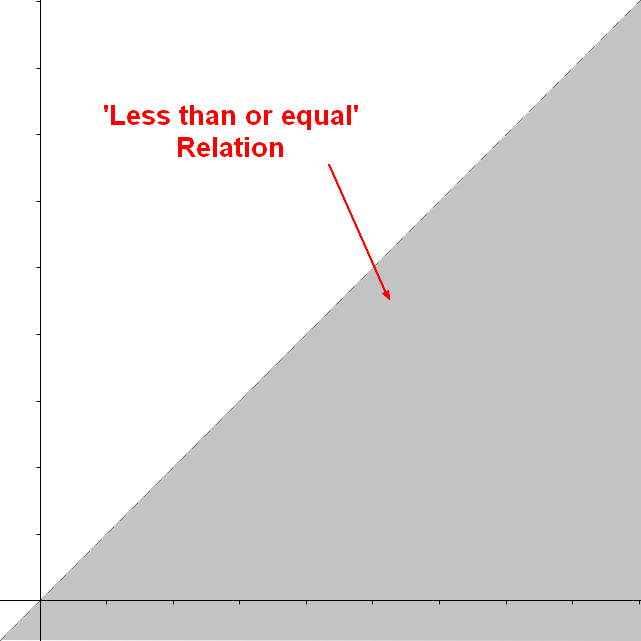# Sort - Less than Operator

less than is a comparison operator that creates a binary relationship.

## Representation

### Set of tuples

The relation less than or equal to (<=) on the set of integers {1, 2, 3} is the following set of tuple

<1, 1>,
<1, 2>,
<1, 3>,
<2, 2>,
<2, 3>,
<3, 3>



### Visual

The relationship less than or equal between y and x (ie y <= x) can be represented in the set $R$ against $R^2$ as the below gray area:

Recommended PagesFunction - Binary Function/Operation

A binary operation is an scalar operation with two arguments (arity of two) that produces one value. They are creating a binary relation. the addition operator, the multiplication operator ...Logical Data Modeling - Reflexive relationship property

reflexive is a relationship property that indicates: that the relationship relates every element to itself. in other word, that the relation set representing the relationship contains every tuple:...Logical Data Modeling - Relationship Representation / Implementation / Visualisation

A relationship can be described/implemented by: a function a a a a A relationship can be represented as a function Example with a three element (ternary) relationship: A binary...Logical Data Modeling - Transitive Relationship Property

transitive is a relationship property that tells that the relationship follows the following rule: when the relation relates a to b and b to c, then the relation relates a to c. In mathematical notation,...Ordinal Data - Comparison Operator (Order, Equality)

A comparison operator is a class of operators that model: a order or a equivalence relationship A comparison operator test the order relationship between two operands and returns a Boolean. The...
What is XML / HTML Character Entity encoding ?

What is XML / HTML Character Entity encoding ? HTML An Entity in html is a string that represents a unicode character. unicode character Example with the 0260Ephone. This character has the unicode...